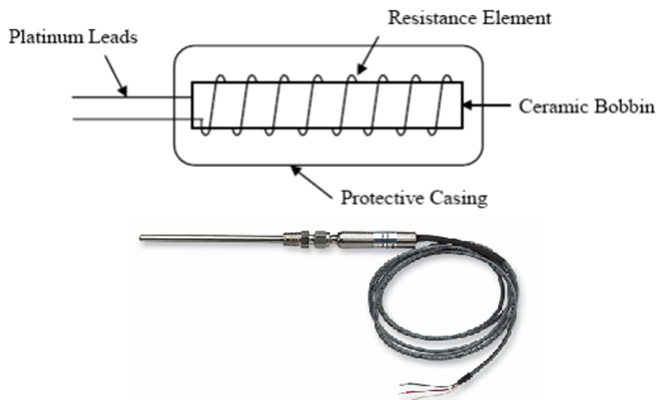Temperature Measurement

# What is RTD? What is the difference between 2 wire, 3 wire, and 4 wire RTD?### What is RTD?

An RTD can be used to detect the temperature, it would vary its resistive property according to the temperature variations. Basically, it is a wire which would be wrapped around a glass core. The major advantage of an RTD is that it is really compact and due to this it can be used for most temperature measurement applications where space is limited. So the resistance temperature detector would determine the change in temperature by the change in the resistance of the wire.

### How is an RTD constructed and how does it work?

The RTDs are mostly made of platinum and its resistance would increase according to the temperature. The resistive property of the material is considered to create an RTD of the given value. Platinum is mostly used for the construction of the RTD because it has higher temperature capability and it provides good stability and repeatability. Platinum is not easily contaminated. So an RTD consist of a probe, resistive material, termination, and lead wires.

The working principle of the RTD is when the metal temperature increases and thus the resistance to the flow of electricity. RTD’s will be provided with electric current, the resistance element in the RTD would be useful to measure the resistance of current which is passed through it. So when the temperature of the resistive element increases then the electrical resistance would increase too.

### What is a 2-wire, 3- wire, and 4-wire RTD and how are they different from each other?

The resistance value of the RTD must be transmitted, RTD measurement is not complete without transmitting the resistance. This resistance value should be transmitted to an instrument, mostly copper wires are used to transmit the RTD resistance to the measuring instrument. These copper wires would have their own resistance, this resistance would be added to the measured RTD resistance. If the wire compensation is not proper then it would create many errors. So to avoid this error various types of compensation are provided with various types of wire connection like 3-wire and 4-wire.

Different types of RTD wiring configuration

2-wire RTD

In this type of configuration, one lead wire will be attached to both sides of the element. The resistance which causes the increase in voltage is the sensor’s total resistance and also there will be resistance from the lead wires. So till the lead wire resistance remains constant it won’t affect the measurement. The resistance of the wire would change with temperature and also because of the variations in the ambient condition. Due to the change in the wire resistance, there would be errors. So if the length of the wire is long then the error would be high. So the two-wire RTDs should be used with short wires.

3 -wire RTD

In this type of configuration three lead wires would come from the RTD. So in this three-wire configuration, two wires would carry the measuring current, while the remaining wire would act as a potential lead. In this type, an additional wire will be added to one contact of the RTD. In a three-wire RTD both sides would be connected with one lead wire and also one extra wire would be connected to one of the sides. The extra wire which is used should be similar to the lead wire which is used in the RTD, like similar length and resistance. So due to this both the wires would have similar resistance and they would cancel each other.

4- wire RTD

The 4-wire configuration can be considered as the most accurate RTD measurement. The constant current would be carried out through two leads, while the remaining two are used to measure the voltage drop. So this type of configuration would remove the error which is caused by the mismatched resistance.

### When should we use an RTD?

• It can be used when accuracy and stability is an important factor
• It can be used when accuracy is important for a wide temperature range
• It can be used when a high degree of standardization is required
• It can be used when area rather than point sensing improves the control

### What are the major advantages of RTD?

• This device doesn’t use fluids for the measurement and due to this it can be used to do the measurement of absolute temperature
• High sensitivity and accurate measurement
• Good range of temperature measurement and due to this it can be used to measure very low to high temperature
• The output is electric and because of this it is suitable to use with PLC

### What are the disadvantages of RTD?

• Sensitivity is low
• Costlier than a thermocouple
• There is no point-sensing
• It would need three or four-wire operation

### What are the applications of RTD?

• It is used in furnaces
• Medical field
• It is used in chemical plants
• Textile industry
• Food industry

RTD can be used for the automatic temperature control of

• Test chambers
• Oven temperatures
• Plastic extruders
• Injection molders
• Solder pots
• Bearing temperature